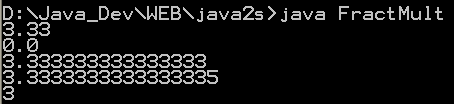# double « Data Type « Java

1.Double class creates primitives that wrap themselves around data items of the double data type
2.Min and Max values of data type double
3.Java double: double is 64 bit double precision type and used when fractional precision calculation is required.
4.Use toString method of Double class to convert Double into String.
5.Use Double constructor to convert double primitive type to a Double object.
6.Convert java Double to numeric primitive data types
7.Convert Java String to Double
8.Java Double compare example
9.Create a Double object using one of the below given constructors
10.Java Double isInfinite
11.Java Double isNaN method
12.Compare Two Java double Arrays
13.Convert from double to String
14.Convert from String to double
15.Converting a String to a double type Number
16.Ternary operator on double value
17.Binary search
18.Linear Searching double Arrays
19.The Circle Area Calculator
20.A Program That Uses the Mathematical Methods of the Math Class
21.A Program That Uses the Rounding Methods of the Math Class
22. Get Double Number
23.Compute the value of 2/3 of 524.Show INFINITY and NaN
25.Double Array
26.Obtaining the integer and fractional parts
27.Get the next machine representable number after a number, moving in the direction of another number.
28.Returns the sign for double precision x
29.Gets the maximum of three double values.
30.Gets the minimum of three double values.
31.Compare two double values with Double.doubleToLongBits
32.Converts altitude in meters to pressure in msw